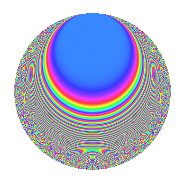# Properties

 Label 861.2.aLevel 861 Weight 2 Character orbit a Rep. character $$\chi_{861}(1,\cdot)$$ Character field $$\Q$$ Dimension 39 Newforms 13 Sturm bound 224 Trace bound 5

# Related objects

## Defining parameters

 Level: $$N$$ = $$861 = 3 \cdot 7 \cdot 41$$ Weight: $$k$$ = $$2$$ Character orbit: $$[\chi]$$ = 861.a (trivial) Character field: $$\Q$$ Newforms: $$13$$ Sturm bound: $$224$$ Trace bound: $$5$$ Distinguishing $$T_p$$: $$2$$, $$5$$

## Dimensions

The following table gives the dimensions of various subspaces of $$M_{2}(\Gamma_0(861))$$.

Total New Old
Modular forms 116 39 77
Cusp forms 109 39 70
Eisenstein series 7 0 7

The following table gives the dimensions of the cuspidal new subspaces with specified eigenvalues for the Atkin-Lehner operators and the Fricke involution.

$$3$$$$7$$$$41$$FrickeDim.
$$+$$$$+$$$$+$$$$+$$$$4$$
$$+$$$$+$$$$-$$$$-$$$$5$$
$$+$$$$-$$$$+$$$$-$$$$8$$
$$+$$$$-$$$$-$$$$+$$$$3$$
$$-$$$$+$$$$+$$$$-$$$$5$$
$$-$$$$+$$$$-$$$$+$$$$4$$
$$-$$$$-$$$$+$$$$+$$$$3$$
$$-$$$$-$$$$-$$$$-$$$$7$$
Plus space$$+$$$$14$$
Minus space$$-$$$$25$$

## Trace form

 $$39q$$ $$\mathstrut +\mathstrut q^{2}$$ $$\mathstrut -\mathstrut q^{3}$$ $$\mathstrut +\mathstrut 45q^{4}$$ $$\mathstrut -\mathstrut 6q^{5}$$ $$\mathstrut +\mathstrut 5q^{6}$$ $$\mathstrut +\mathstrut 3q^{7}$$ $$\mathstrut -\mathstrut 3q^{8}$$ $$\mathstrut +\mathstrut 39q^{9}$$ $$\mathstrut +\mathstrut O(q^{10})$$ $$39q$$ $$\mathstrut +\mathstrut q^{2}$$ $$\mathstrut -\mathstrut q^{3}$$ $$\mathstrut +\mathstrut 45q^{4}$$ $$\mathstrut -\mathstrut 6q^{5}$$ $$\mathstrut +\mathstrut 5q^{6}$$ $$\mathstrut +\mathstrut 3q^{7}$$ $$\mathstrut -\mathstrut 3q^{8}$$ $$\mathstrut +\mathstrut 39q^{9}$$ $$\mathstrut -\mathstrut 10q^{10}$$ $$\mathstrut -\mathstrut 4q^{11}$$ $$\mathstrut -\mathstrut 7q^{12}$$ $$\mathstrut -\mathstrut 14q^{13}$$ $$\mathstrut +\mathstrut q^{14}$$ $$\mathstrut +\mathstrut 2q^{15}$$ $$\mathstrut +\mathstrut 45q^{16}$$ $$\mathstrut -\mathstrut 10q^{17}$$ $$\mathstrut +\mathstrut q^{18}$$ $$\mathstrut +\mathstrut 4q^{19}$$ $$\mathstrut -\mathstrut 2q^{20}$$ $$\mathstrut -\mathstrut q^{21}$$ $$\mathstrut +\mathstrut 20q^{22}$$ $$\mathstrut +\mathstrut 8q^{23}$$ $$\mathstrut +\mathstrut 9q^{24}$$ $$\mathstrut +\mathstrut 9q^{25}$$ $$\mathstrut +\mathstrut 14q^{26}$$ $$\mathstrut -\mathstrut q^{27}$$ $$\mathstrut +\mathstrut 5q^{28}$$ $$\mathstrut -\mathstrut 6q^{29}$$ $$\mathstrut -\mathstrut 10q^{30}$$ $$\mathstrut +\mathstrut 20q^{31}$$ $$\mathstrut +\mathstrut 5q^{32}$$ $$\mathstrut -\mathstrut 8q^{33}$$ $$\mathstrut -\mathstrut 22q^{34}$$ $$\mathstrut -\mathstrut 6q^{35}$$ $$\mathstrut +\mathstrut 45q^{36}$$ $$\mathstrut -\mathstrut 10q^{37}$$ $$\mathstrut +\mathstrut 12q^{38}$$ $$\mathstrut +\mathstrut 2q^{39}$$ $$\mathstrut +\mathstrut 30q^{40}$$ $$\mathstrut -\mathstrut q^{41}$$ $$\mathstrut -\mathstrut 3q^{42}$$ $$\mathstrut -\mathstrut 32q^{43}$$ $$\mathstrut +\mathstrut 20q^{44}$$ $$\mathstrut -\mathstrut 6q^{45}$$ $$\mathstrut +\mathstrut 24q^{46}$$ $$\mathstrut -\mathstrut 24q^{47}$$ $$\mathstrut +\mathstrut q^{48}$$ $$\mathstrut +\mathstrut 39q^{49}$$ $$\mathstrut +\mathstrut 31q^{50}$$ $$\mathstrut +\mathstrut 2q^{51}$$ $$\mathstrut -\mathstrut 66q^{52}$$ $$\mathstrut -\mathstrut 14q^{53}$$ $$\mathstrut +\mathstrut 5q^{54}$$ $$\mathstrut -\mathstrut 16q^{55}$$ $$\mathstrut +\mathstrut 21q^{56}$$ $$\mathstrut -\mathstrut 4q^{57}$$ $$\mathstrut +\mathstrut 54q^{58}$$ $$\mathstrut +\mathstrut 4q^{59}$$ $$\mathstrut +\mathstrut 6q^{60}$$ $$\mathstrut +\mathstrut 6q^{61}$$ $$\mathstrut -\mathstrut 40q^{62}$$ $$\mathstrut +\mathstrut 3q^{63}$$ $$\mathstrut +\mathstrut 45q^{64}$$ $$\mathstrut -\mathstrut 28q^{65}$$ $$\mathstrut +\mathstrut 12q^{66}$$ $$\mathstrut +\mathstrut 4q^{67}$$ $$\mathstrut -\mathstrut 38q^{68}$$ $$\mathstrut +\mathstrut 8q^{69}$$ $$\mathstrut +\mathstrut 14q^{70}$$ $$\mathstrut +\mathstrut 32q^{71}$$ $$\mathstrut -\mathstrut 3q^{72}$$ $$\mathstrut -\mathstrut 38q^{73}$$ $$\mathstrut -\mathstrut 50q^{74}$$ $$\mathstrut -\mathstrut 15q^{75}$$ $$\mathstrut +\mathstrut 12q^{76}$$ $$\mathstrut +\mathstrut 20q^{77}$$ $$\mathstrut -\mathstrut 18q^{78}$$ $$\mathstrut +\mathstrut 32q^{79}$$ $$\mathstrut -\mathstrut 50q^{80}$$ $$\mathstrut +\mathstrut 39q^{81}$$ $$\mathstrut -\mathstrut 3q^{82}$$ $$\mathstrut +\mathstrut 92q^{83}$$ $$\mathstrut -\mathstrut 7q^{84}$$ $$\mathstrut -\mathstrut 4q^{85}$$ $$\mathstrut -\mathstrut 36q^{86}$$ $$\mathstrut -\mathstrut 26q^{87}$$ $$\mathstrut +\mathstrut 4q^{88}$$ $$\mathstrut +\mathstrut 38q^{89}$$ $$\mathstrut -\mathstrut 10q^{90}$$ $$\mathstrut -\mathstrut 14q^{91}$$ $$\mathstrut -\mathstrut 24q^{92}$$ $$\mathstrut -\mathstrut 24q^{93}$$ $$\mathstrut +\mathstrut 48q^{94}$$ $$\mathstrut +\mathstrut 40q^{95}$$ $$\mathstrut +\mathstrut 33q^{96}$$ $$\mathstrut -\mathstrut 18q^{97}$$ $$\mathstrut +\mathstrut q^{98}$$ $$\mathstrut -\mathstrut 4q^{99}$$ $$\mathstrut +\mathstrut O(q^{100})$$

## Decomposition of $$S_{2}^{\mathrm{new}}(\Gamma_0(861))$$ into irreducible Hecke orbits

Label Dim. $$A$$ Field CM Traces A-L signs $q$-expansion
$$a_2$$ $$a_3$$ $$a_5$$ $$a_7$$ 3 7 41
861.2.a.a $$1$$ $$6.875$$ $$\Q$$ None $$-1$$ $$-1$$ $$2$$ $$1$$ $$+$$ $$-$$ $$+$$ $$q-q^{2}-q^{3}-q^{4}+2q^{5}+q^{6}+q^{7}+\cdots$$
861.2.a.b $$1$$ $$6.875$$ $$\Q$$ None $$-1$$ $$1$$ $$-3$$ $$1$$ $$-$$ $$-$$ $$+$$ $$q-q^{2}+q^{3}-q^{4}-3q^{5}-q^{6}+q^{7}+\cdots$$
861.2.a.c $$1$$ $$6.875$$ $$\Q$$ None $$-1$$ $$1$$ $$3$$ $$-1$$ $$-$$ $$+$$ $$-$$ $$q-q^{2}+q^{3}-q^{4}+3q^{5}-q^{6}-q^{7}+\cdots$$
861.2.a.d $$1$$ $$6.875$$ $$\Q$$ None $$1$$ $$1$$ $$-1$$ $$-1$$ $$-$$ $$+$$ $$-$$ $$q+q^{2}+q^{3}-q^{4}-q^{5}+q^{6}-q^{7}+\cdots$$
861.2.a.e $$2$$ $$6.875$$ $$\Q(\sqrt{2})$$ None $$-2$$ $$2$$ $$-2$$ $$2$$ $$-$$ $$-$$ $$+$$ $$q+(-1+\beta )q^{2}+q^{3}+(1-2\beta )q^{4}-q^{5}+\cdots$$
861.2.a.f $$2$$ $$6.875$$ $$\Q(\sqrt{17})$$ None $$-1$$ $$-2$$ $$4$$ $$2$$ $$+$$ $$-$$ $$+$$ $$q-\beta q^{2}-q^{3}+(2+\beta )q^{4}+2q^{5}+\beta q^{6}+\cdots$$
861.2.a.g $$2$$ $$6.875$$ $$\Q(\sqrt{17})$$ None $$-1$$ $$2$$ $$-3$$ $$-2$$ $$-$$ $$+$$ $$-$$ $$q-\beta q^{2}+q^{3}+(2+\beta )q^{4}+(-2+\beta )q^{5}+\cdots$$
861.2.a.h $$3$$ $$6.875$$ 3.3.148.1 None $$-1$$ $$-3$$ $$1$$ $$3$$ $$+$$ $$-$$ $$-$$ $$q-\beta _{1}q^{2}-q^{3}+(\beta _{1}+\beta _{2})q^{4}+(\beta _{1}+\beta _{2})q^{5}+\cdots$$
861.2.a.i $$4$$ $$6.875$$ 4.4.8468.1 None $$1$$ $$-4$$ $$-3$$ $$-4$$ $$+$$ $$+$$ $$+$$ $$q+\beta _{1}q^{2}-q^{3}+(1+\beta _{2})q^{4}+(-1-\beta _{2}+\cdots)q^{5}+\cdots$$
861.2.a.j $$5$$ $$6.875$$ 5.5.981328.1 None $$-3$$ $$-5$$ $$1$$ $$-5$$ $$+$$ $$+$$ $$-$$ $$q+(-1+\beta _{1})q^{2}-q^{3}+(2-\beta _{1}+\beta _{2}+\cdots)q^{4}+\cdots$$
861.2.a.k $$5$$ $$6.875$$ 5.5.1197392.1 None $$3$$ $$-5$$ $$-9$$ $$5$$ $$+$$ $$-$$ $$+$$ $$q+(1-\beta _{1})q^{2}-q^{3}+(2+\beta _{2})q^{4}+(-1+\cdots)q^{5}+\cdots$$
861.2.a.l $$5$$ $$6.875$$ 5.5.626512.1 None $$3$$ $$5$$ $$3$$ $$-5$$ $$-$$ $$+$$ $$+$$ $$q+(1-\beta _{1})q^{2}+q^{3}+(2-\beta _{1}-\beta _{2}+\beta _{3}+\cdots)q^{4}+\cdots$$
861.2.a.m $$7$$ $$6.875$$ $$\mathbb{Q}[x]/(x^{7} - \cdots)$$ None $$4$$ $$7$$ $$1$$ $$7$$ $$-$$ $$-$$ $$-$$ $$q+(1-\beta _{1})q^{2}+q^{3}+(2-\beta _{1}+\beta _{2})q^{4}+\cdots$$

## Decomposition of $$S_{2}^{\mathrm{old}}(\Gamma_0(861))$$ into lower level spaces

$$S_{2}^{\mathrm{old}}(\Gamma_0(861)) \cong$$ $$S_{2}^{\mathrm{new}}(\Gamma_0(21))$$$$^{\oplus 2}$$$$\oplus$$$$S_{2}^{\mathrm{new}}(\Gamma_0(41))$$$$^{\oplus 4}$$$$\oplus$$$$S_{2}^{\mathrm{new}}(\Gamma_0(123))$$$$^{\oplus 2}$$$$\oplus$$$$S_{2}^{\mathrm{new}}(\Gamma_0(287))$$$$^{\oplus 2}$$